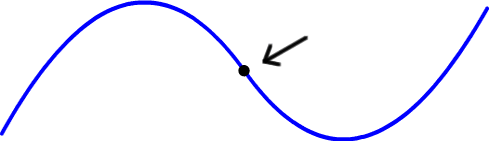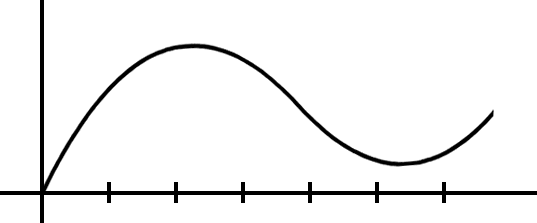One of the other things you'll be hunting for is the point where the graph changes from concave down to concave up (and visa versa).It's called "the point of inflection."

This tangent line stuff was probably way more than you wanted to know.  But, believe me, it's nice to get to see it all ahead of time before you have all those Calculus numbers, x's and formulas flying at you at what will seem like the speed of light!

YOUR TURN:

On the following graph, draw tangent lines at
x = 1, x = 2, x = 3 , x = 4, x = 5 and x = 6.Where is the graph concave down?

Where is the graph concave up?